Share filter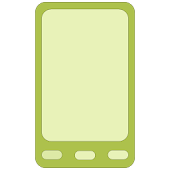Developed by in category

4/5.0 Ratings

5,000 - 10,000 Installs

36 Reviews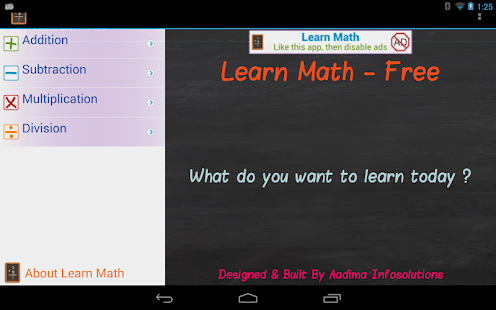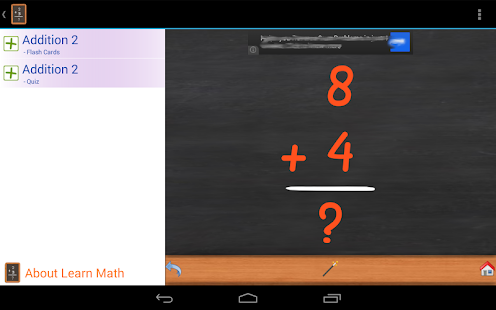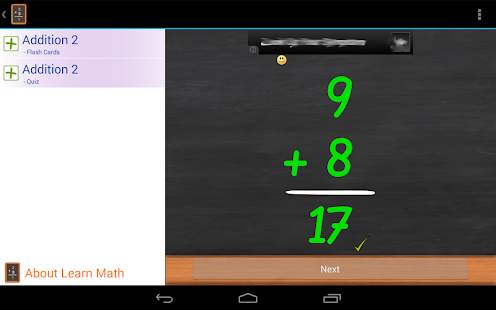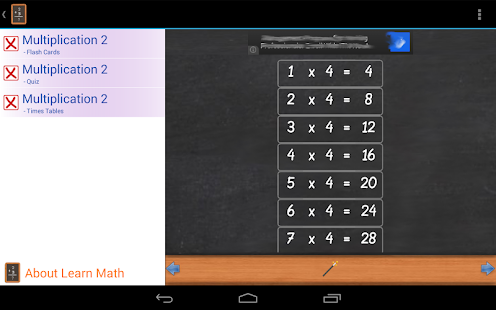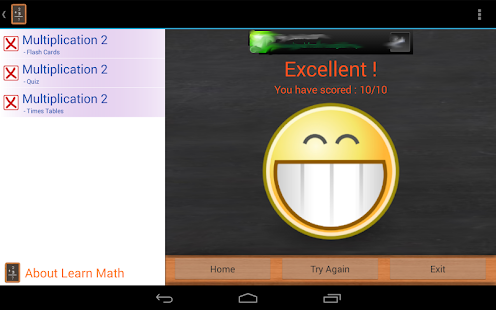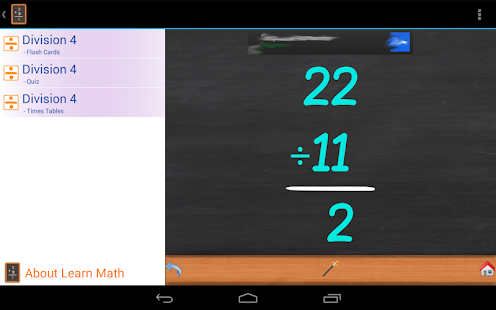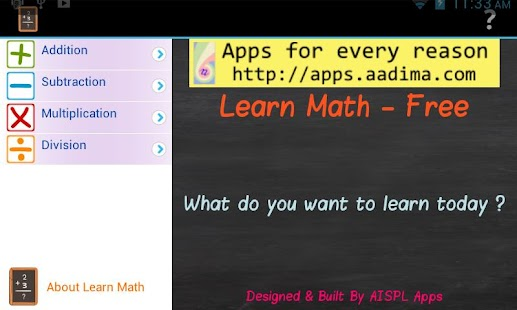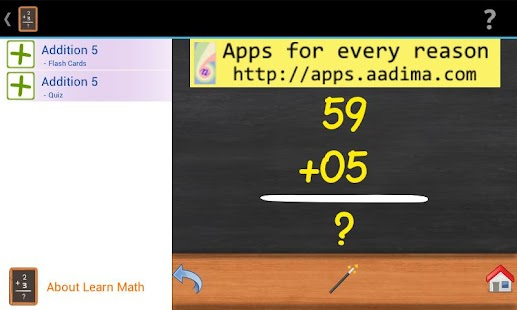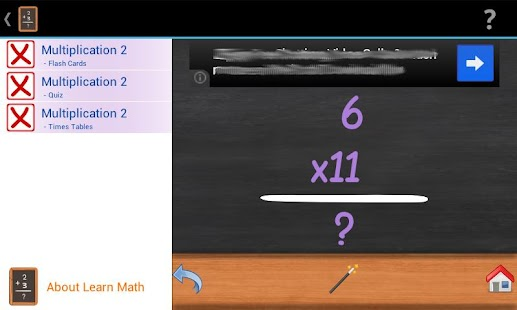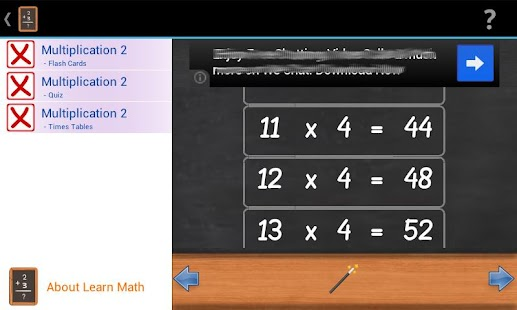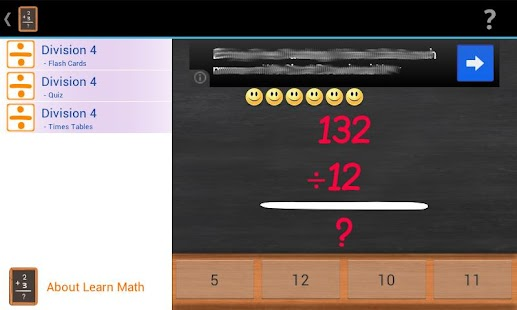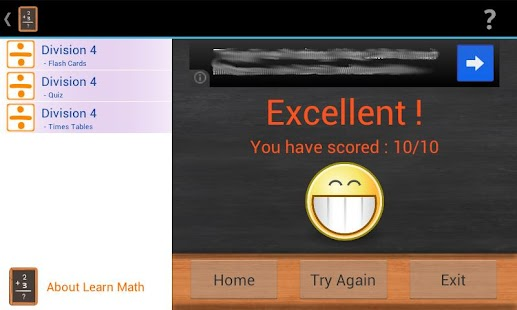### Description

Learn Math - Free with Ads

Learn Math is for Kids who are starting to learn math !. Kids can learn to add, subtract, multiply and divide. The app introduces these concepts in stages of increasing complexity. Kids can practice and pick up these skills at their own pace.
All the stages in the app have flash cards and practice quiz and includes times tables for multiplication and division.
Addition is divided into 7 stages for easy learning, each stage adds to the previous stages. These stages are
Stage 1 - Single Digit Addition upto 10
Stage 2 - Single Digit Addition upto 18
Stage 3 - Add one digit number to a 2 digit number without regrouping (without carrying)
Stage 4 - Add 2 digit number to a 2 digit number without regrouping (without carrying)
Stage 5 - Add one digit number to a 2 digit number with regrouping (with carrying)
Stage 6 - Add 2 digit number to a 2 digit number with regrouping (with carrying)
Stage 7 - Adding 3 digit numbers
Subtraction
Subtraction is divided into 7 stages for easy learning, each stage adds to the previous stages. These stages are
Stage 1 - Single Digit Subtraction upto 10
Stage 2 - Single Digit Subtraction upto 18
Stage 3 - Subtract one digit number to a 2 digit number without regrouping (without borrowing)
Stage 4 - Subtract 2 digit number to a 2 digit number without regrouping (without borrowing)
Stage 5 - Subtract one digit number to a 2 digit number with regrouping (with borrowing)
Stage 6 - Subtract 2 digit number to a 2 digit number with regrouping (with borrowing)
Stage 7 - Subtracting 3 digit numbers
Multiplication
Multiplication has 4 stages for easy learning each containing 3 times tables.
Stage 1 - Times Tables upto 3
Stage 2 - Times Tables upto 6
Stage 3 - Times Tables upto 9
Stage 4 - Times Tables upto 12
Division
Division has 4 stages for easy learning each containing 3 times tables.
Stage 1 - Times Tables upto 3
Stage 2 - Times Tables upto 6
Stage 3 - Times Tables upto 9
Stage 4 - Times Tables upto 12
This App default installs on SD Card.
Keywords: Kids, Educational, Child, Children, Learn, Learning, Basic, Skills, Games, School, Kindergarten,
Numbers, Math, Maths, Add, Subtract, Multiply, Divide, Addition, Subtraction, Multiplication, Division, Times Tables, Quiz, Flash Cards, Arithmetic, Study Aid, Beginners, Learners, practice quizzes, single digit to 3 digits, easy-to-use , intuitive.

This content has been crawled through Play Store, we don't store any APK file.